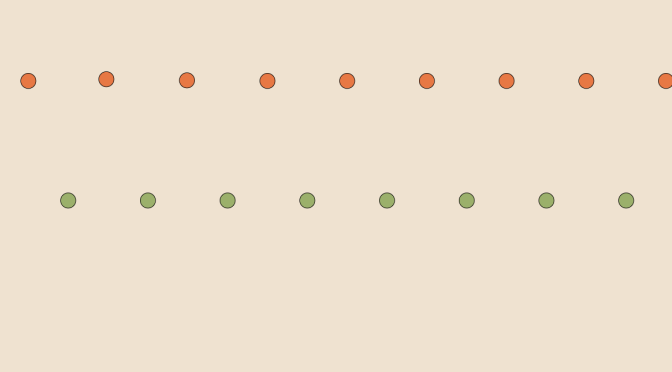# Counterexamples on real sequences (part 1)

I will come back later on with more complex cases. Unless otherwise stated, $$(u_n)_{n \in \mathbb{N}}$$ and $$(v_n)_{n \in \mathbb{N}}$$ are two real sequences.

#### If $$\lim u_n = 0$$ then $$\lim u_n v_n = 0$$?

Is not true. Taking $$u_n = \frac{1}{n+1}$$ and $$v_n = n+1$$ we get $$u_n v_n = 1$$ for all $$n$$. We can even get $$\lim (u_n v_n) = + \infty$$ for $$u_n = \frac{1}{n+1}$$ and $$v_n = (n+1)^2$$.

#### If $$\lim (u_n v_n) = 0$$ then $$\lim u_n= 0$$ or $$\lim v_n= 0$$?

Does not hold as can be seen by taking $$u_n=0$$ for $$n$$ even and $$u_n=1$$ for $$n$$ odd, while taking $$v_n=1$$ for $$n$$ even and $$v_n=0$$ for $$n$$ odd.
With following other example: $$u_n=\begin{cases} 0 & \text{for } n \text{ even} \\ n & \text{for } n \text{ odd} \end{cases}$$ and
$$v_n=\begin{cases} n & \text{for } n \text{ even} \\ 0 & \text{for } n \text{ odd} \end{cases}$$ we even have $$u_n v_n = 0$$ for all $$n$$ while $$(u_n)$$ and $$(v_n)$$ are both unbounded.

#### If $$\lim (u_n + v_n) = + \infty$$ then $$\lim u_n= + \infty$$ or $$\lim v_n= + \infty$$?

Is not true as we can see with the sequences of previous question, i.e.
$$u_n=\begin{cases} 0 & \text{for } n \text{ even} \\ n & \text{for } n \text{ odd} \end{cases}$$ and
$$v_n=\begin{cases} n & \text{for } n \text{ even} \\ 0 & \text{for } n \text{ odd.} \end{cases}$$ However one of the sequence has to be unbounded as the sum of two bounded sequences is bounded.

#### If $$(u_n)$$ is a strictly decreasing sequence of positive reals then $$\lim u_n = 0$$?

Absolutely not! Consider $$u_n=1+\frac{1}{n+1}$$.

#### If $$(u_n)$$ is a positive real sequence and $$\lim u_n = 0$$ then $$(u_n)$$ is eventually decreasing?

Not either. Have a look at $$u_n=\begin{cases} \frac{1}{n+1} & \text{for } n \text{ even} \\ \frac{1}{(n+1)^2} & \text{for } n \text{ odd.} \end{cases}$$

#### If $$u_n > 0$$ for all integers then $$\lim (n u_n) = + \infty$$?

Still not… For $$u_n = \frac{1}{(n+1)^2}$$ we have $$\lim (n u_n) = 0$$.

#### If $$u_n > 1$$ for all integers then $$\lim (u_n)^n = + \infty$$?

Is not true. The sequence $$u_n = 1 + \frac{1}{n}$$ for $$n \ge 1$$ and $$u_0=0$$ is such that $$\displaystyle \lim\limits_{n \to +\infty} (u_n)^n = \lim\limits_{n \to +\infty} (1 + \frac{1}{n})^n = e$$. Where $$e$$ is the well known e (mathematical constant).

#### If $$\lim (u_{n+1}-u_n) =0$$ then $$(u_n)$$ is convergent?

Sorry to provide again a negative answer – the last one! The harmonic series $$u_n = \displaystyle \sum_{k=0}^n \frac{1}{k+1}$$ diverges.